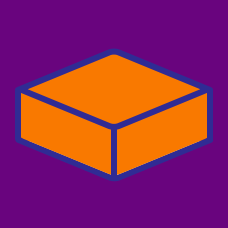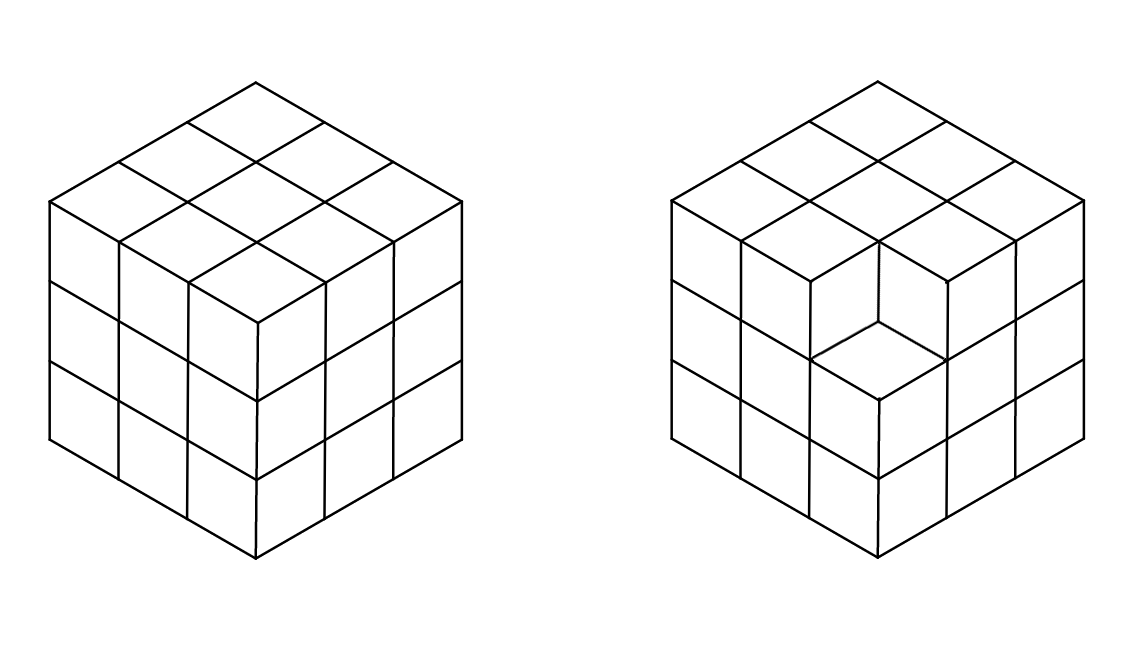Geometry

Surface Area Warmup

27 cubes are stacked to create a larger 3-by-3 cube. If one corner cube is removed, how will the surface area of the figure change?You need to wrap a cube-shaped box before mailing it. The box's edge length is 2 feet. How many square feet of wrapping paper do you need to completely wrap the box?

If the side lengths of a cube double, what happens to the cube's surface area?

Is there more than one combination of integer dimensions that will create a surface area of 22 on a cuboid?

If you glued together four cube-shaped boxes, each having a side length of one foot, what is the maximum surface area this new shape could have? Any glued side must be completely covered and does not count towards total surface area.

×